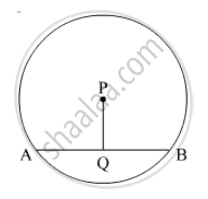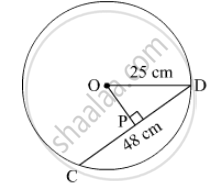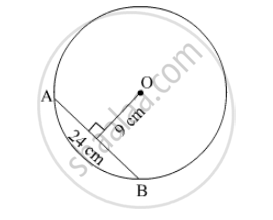# Question Bank Solutions for SSC (English Medium) 8th Standard - Maharashtra State Board - Mathematics

Subjects
Topics
Subjects
Popular subjects
Topics
Mathematics
< prev  21 to 40 of 360  next >

Show the following numbers on a number line. Draw a separate number line for each example.

7/5 , (-2)/5 , (-4)/5

[0.01] Rational and Irrational Numbers
Chapter: [0.01] Rational and Irrational Numbers
Concept: Rational Numbers on a Number Line

Show the following numbers on a number line. Draw a separate number line for each example.

(-5)/8 , 11/8

[0.01] Rational and Irrational Numbers
Chapter: [0.01] Rational and Irrational Numbers
Concept: Rational Numbers on a Number Line

Show the following numbers on a number line. Draw a separate number line for each example.

13/10 , (-17)/10

[0.01] Rational and Irrational Numbers
Chapter: [0.01] Rational and Irrational Numbers
Concept: Rational Numbers on a Number Line

The number sqrt2 is shown on a number line. Steps are given to show sqrt3 on the number line using sqrt2. Fill in the boxes properly and complete the activity.

Activity :

• The point Q on the number line shows the number ...... .
• A line perpendicular to the number line is drawn through the point Q. Point R is at unit distance from Q on the line.
• Right angled  ORQ is obtained by drawing seg OR.

l  (OQ) = sqrt2 ,    l(QR) = 1

therefore by Pythagoras theorem,

[l(OR)]^2  = [l(OQ)]^2 + [l(QR)]^2

= _____2 + _____2 = _____ + _____

= ______

∴ l(OR) = _____

Draw an arc with centre O and radius OR. Mark the point of intersection of the line and the arc as C. The point C shows the number √3.

[0.01] Rational and Irrational Numbers
Chapter: [0.01] Rational and Irrational Numbers
Concept: Rational Numbers on a Number Line

Show the number √5 on the number line.

[0.01] Rational and Irrational Numbers
Chapter: [0.01] Rational and Irrational Numbers
Concept: Rational Numbers on a Number Line

Show the number √7 on the number line.

[0.01] Rational and Irrational Numbers
Chapter: [0.01] Rational and Irrational Numbers
Concept: Rational Numbers on a Number Line

Expand.

(a+2)(a−1)

[0.05] Expansion Formulae
Chapter: [0.05] Expansion Formulae
Concept: Expansion of (x + a)(x + b)

Expand.

(m−4)(m+6)

[0.05] Expansion Formulae
Chapter: [0.05] Expansion Formulae
Concept: Expansion of (x + a)(x + b)

Expand.

(p+8) (p−3)

[0.05] Expansion Formulae
Chapter: [0.05] Expansion Formulae
Concept: Expansion of (x + a)(x + b)

Expand.

(13+x) (13−x)

[0.05] Expansion Formulae
Chapter: [0.05] Expansion Formulae
Concept: Expansion of (x + a)(x + b)

Expand.

(3x+4y) (3x+5y)

[0.05] Expansion Formulae
Chapter: [0.05] Expansion Formulae
Concept: Expansion of (x + a)(x + b)

Expand.

(9x−5t) (9x+3t)

[0.05] Expansion Formulae
Chapter: [0.05] Expansion Formulae
Concept: Expansion of (x + a)(x + b)

In a circle with centre P, chord AB is drawn of length 13 cm, seg PQ ⊥ chord AB, then find l(QB).[0.17] Circle - Chord and Arc
Chapter: [0.17] Circle - Chord and Arc
Concept: Properties of Chord of a Circle

Expand.

(m+2/3)(m-7/3)

[0.05] Expansion Formulae
Chapter: [0.05] Expansion Formulae
Concept: Expansion of (x + a)(x + b)

Expand.

(x+1/x)(x-1/x)

[0.05] Expansion Formulae
Chapter: [0.05] Expansion Formulae
Concept: Expansion of (x + a)(x + b)

Expand.

(1/y+4)(1/y-9)

[0.05] Expansion Formulae
Chapter: [0.05] Expansion Formulae
Concept: Expansion of (x + a)(x + b)

Radius of a circle with centre O is 25 cm. Find the distance of a chord from the centre if length of the chord is 48 cm.[0.17] Circle - Chord and Arc
Chapter: [0.17] Circle - Chord and Arc
Concept: Properties of Chord of a Circle

O is centre of the circle. Find the length of radius, if the chord of length 24 cm is at a distance of 9 cm from the centre of the circle.[0.17] Circle - Chord and Arc
Chapter: [0.17] Circle - Chord and Arc
Concept: Properties of Chord of a Circle

C is the centre of the circle whose radius is 10 cm. Find the distance of the chord from the centre if the length of the chord is 12 cm.

[0.17] Circle - Chord and Arc
Chapter: [0.17] Circle - Chord and Arc
Concept: Properties of Chord of a Circle

Find the volume of a box if its length, breadth and height are 20 cm, 10.5 cm and 8 cm respectively.

[0.16] Surface Area and Volume
Chapter: [0.16] Surface Area and Volume
Concept: Standard Unit of Volume
< prev  21 to 40 of 360  next >